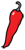The Grand Locus / Life for statistical sciences

the Blog

## Fisher information (with a cat)It is still summer but the days are getting shorter (p < 0.05). Edgar and Sofia are playing chess, Immanuel purrs in a sofa next to them. Edgar has been holding his head for a while, thinking about his next move. Sofia starts:

“Something bothers me Immanuel. In the last post, you told us that Fisher information could be defined as a variance, but that is not what I remember from my classes of mathematical statistics.”

“What do you remember, Sofia?”

“Our teacher said it was the curvature of the log-likelihood function around the maximum. More specifically, consider a parametric model $f(X;\theta)$ where $X$ is a random variable and $\theta$ is a parameter. Say that the true (but unknown) value of the parameter is $\theta^*$. The first terms of the Taylor expansion of the log-likelihood $\log f(X;\theta)$ around $\theta^*$ are

$$\log f(X;\theta^*) + (\theta - \theta^*) \cdot \frac{\partial}{\partial \theta} \log f(X;\theta^*) + \frac{1}{2}(\theta - \theta^*)^2 \cdot \frac{\partial^2}{\partial \theta^2} \log f(X;\theta^*).$$

Now compute the expected value and obtain the approximation below. We call it $\varphi(\theta)$ to emphasize that it is...